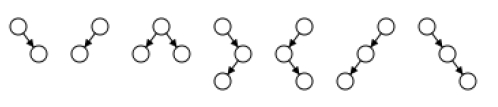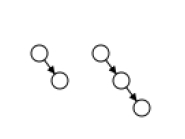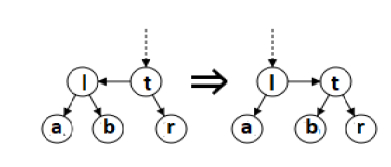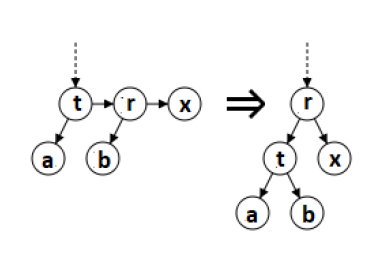# AA Trees in C/C++?

An AA tree in computer science is defined as a form of balanced tree implemented for storing and retrieving ordered data efficiently. AA trees are treated as a variation of the red-black tree, a form of binary search tree which supports efficient addition and deletion of entries. As opposed to red-black trees, red nodes on an AA tree can only be added as a right subchild, no left subchild. The result of this operation is the simulation of a 2-3 tree instead of a 2-3-4 tree, which causes simplification the maintenance operations. The maintenance algorithms for a red-black tree require to assume or consider seven different shapes to properly balance the tree −As opposed to red-black tree, an AA tree on the other hand only requires to assume or consider two shapes due to the strict requirement that only right links can be red −Balancing rotations

Whereas red-black trees need one bit of balancing metadata per node (the color), AA trees need O(log(log(N))) bits of metadata per node, in the form of an integer "level". The below invariants hold for AA trees −

• The level of every leaf node is treated as one.

• The level of every left child is exactly one smaller than that of its parent.

• The level of every right child is equal to or one smaller than that of its parent.

• The level of every right grandchild is strictly smaller than that of its grandparent.

• Every node of level higher than one has two children.

Re-balancing an AA tree is procedurally much easier or simpler than re-balancing a red-black tree.

In case of an AA tree only two distinct operations are required for restoring balance: "skew" and "split". Skew is treated as a right rotation to replace a subtree consisting of a left horizontal link with one consisting of a right horizontal link instead. In case of Split, it is a left rotation and level increase to replace a subtree consisting two or more consecutive right horizontal links with one containing two fewer consecutive right horizontal links. The operations, "skew" and "split" , are explained below −

## function skew is

   input: An AA tree that needs to be rebalanced is represented by a node, t.
output: The rebalanced AA tree is represented by another node.
if nil(t) then
return nil
else if nil(left(t)) then
return t
else if level(left(t)) == level(t) then
Exchange the pointers of horizontal left links.
l = left(t)
left(t) := right(l)
right(l) := t
return l
else
return t
end if
end function## function split is

   input: An AA tree that needs to be rebalanced is represented by a node, t.
output: The rebalanced AA tree is represented by another node.
if nil(t) then
return nil
else if nil(right(t)) or nil(right(right(t))) then
return t
else if level(t) == level(right(right(t))) then
We have two horizontal right links. The middle node is taken, elevate it, and return it.
r = right(t)
right(t) := left(r)
left(r) := t
level(r) := level(r) + 1
return r
else
return t
end if
end functionSplit −

Updated on: 29-Jan-2020

648 Views